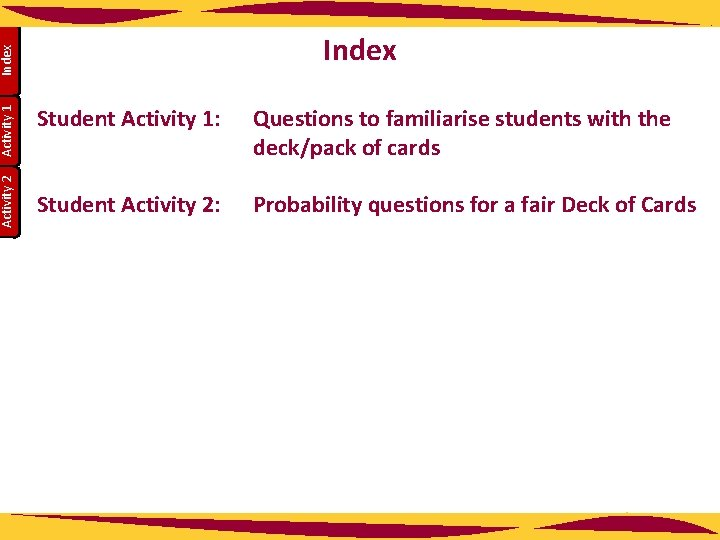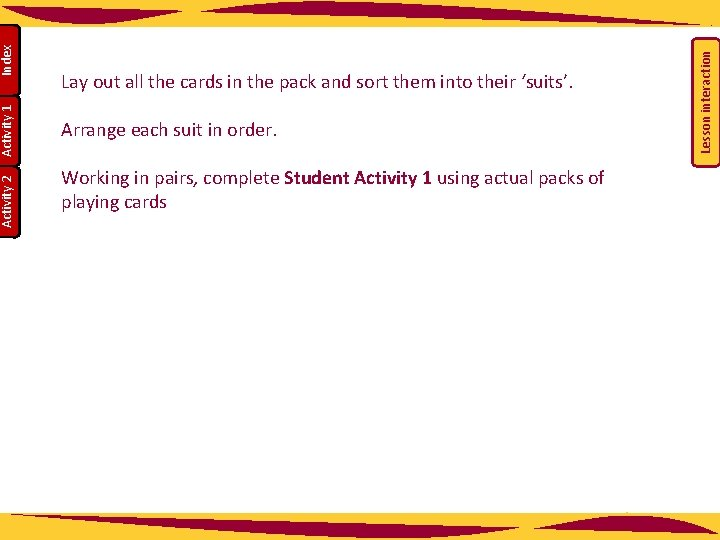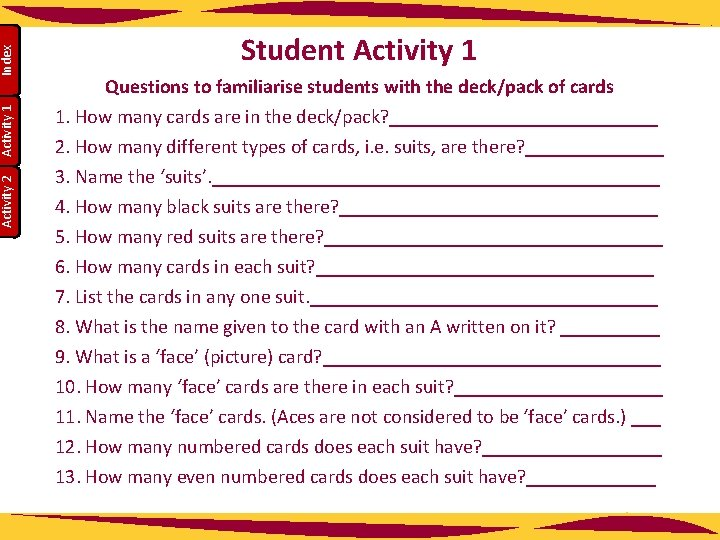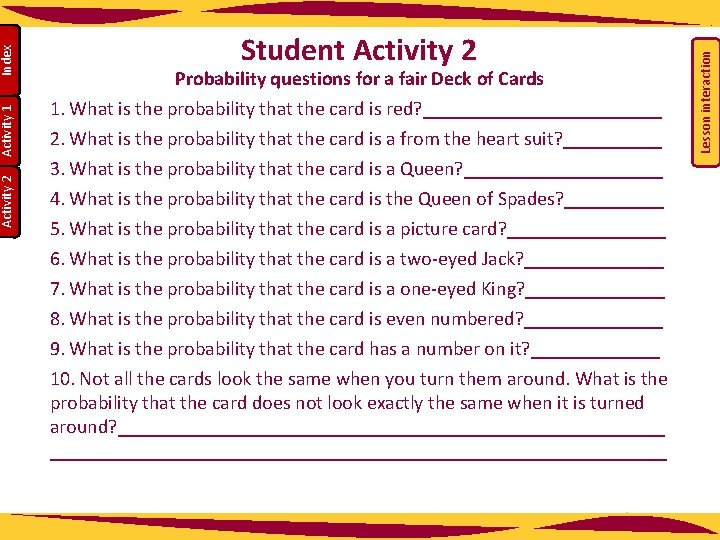# Activity 2 Activity 1 Index Student Activity 1

• Slides: 6Activity 2 Activity 1 Index Student Activity 1: Questions to familiarise students with the deck/pack of cards Student Activity 2: Probability questions for a fair Deck of CardsArrange each suit in order. Working in pairs, complete Student Activity 1 using actual packs of playing cards Lesson interaction Index Activity 1 Activity 2 Lay out all the cards in the pack and sort them into their ‘suits’.Index Activity 1 Activity 2 Student Activity 1 Questions to familiarise students with the deck/pack of cards 1. How many cards are in the deck/pack? ______________ 2. How many different types of cards, i. e. suits, are there? _______ 3. Name the ‘suits’. _______________________ 4. How many black suits are there? ________________ 5. How many red suits are there? _________________ 6. How many cards in each suit? _________________ 7. List the cards in any one suit. __________________ 8. What is the name given to the card with an A written on it? _____ 9. What is a ‘face’ (picture) card? _________________ 10. How many ‘face’ cards are there in each suit? ___________ 11. Name the ‘face’ cards. (Aces are not considered to be ‘face’ cards. ) ___ 12. How many numbered cards does each suit have? _________ 13. How many even numbered cards does each suit have? _______Index Activity 1 Activity 2 14. How many odd numbered cards does each suit have? _______ 15. How many non-numbered (lettered) cards are in each suit? _____ 16. Which card is non-numbered and is not a face (picture) card? ____ 17. How many black cards are there in a fair deck? __________ 18. How many red cards are there in a fair deck? ___________ 19. In the whole deck: a. How many even numbered cards are there? __________ b. How many odd numbered cards are there? __________ c. How many lettered cards are there? ______________ d. How many picture (face) cards are there? ___________ e. How many of each kind of card are there in the full pack? (i. e. how many 1’s, 2’s, …Aces, Kings, etc)? ____________ f. What is the lowest numbered card you can pick in any suit? ____Probability questions for a fair Deck of Cards 1. What is the probability that the card is red? ____________ 2. What is the probability that the card is a from the heart suit? _____ 3. What is the probability that the card is a Queen? __________ 4. What is the probability that the card is the Queen of Spades? _____ 5. What is the probability that the card is a picture card? ________ 6. What is the probability that the card is a two-eyed Jack? _______ 7. What is the probability that the card is a one-eyed King? _______ 8. What is the probability that the card is even numbered? _______ 9. What is the probability that the card has a number on it? _______ 10. Not all the cards look the same when you turn them around. What is the probability that the card does not look exactly the same when it is turned around? ______________________________________________________________ Lesson interaction Index Activity 1 Activity 2 Student Activity 2